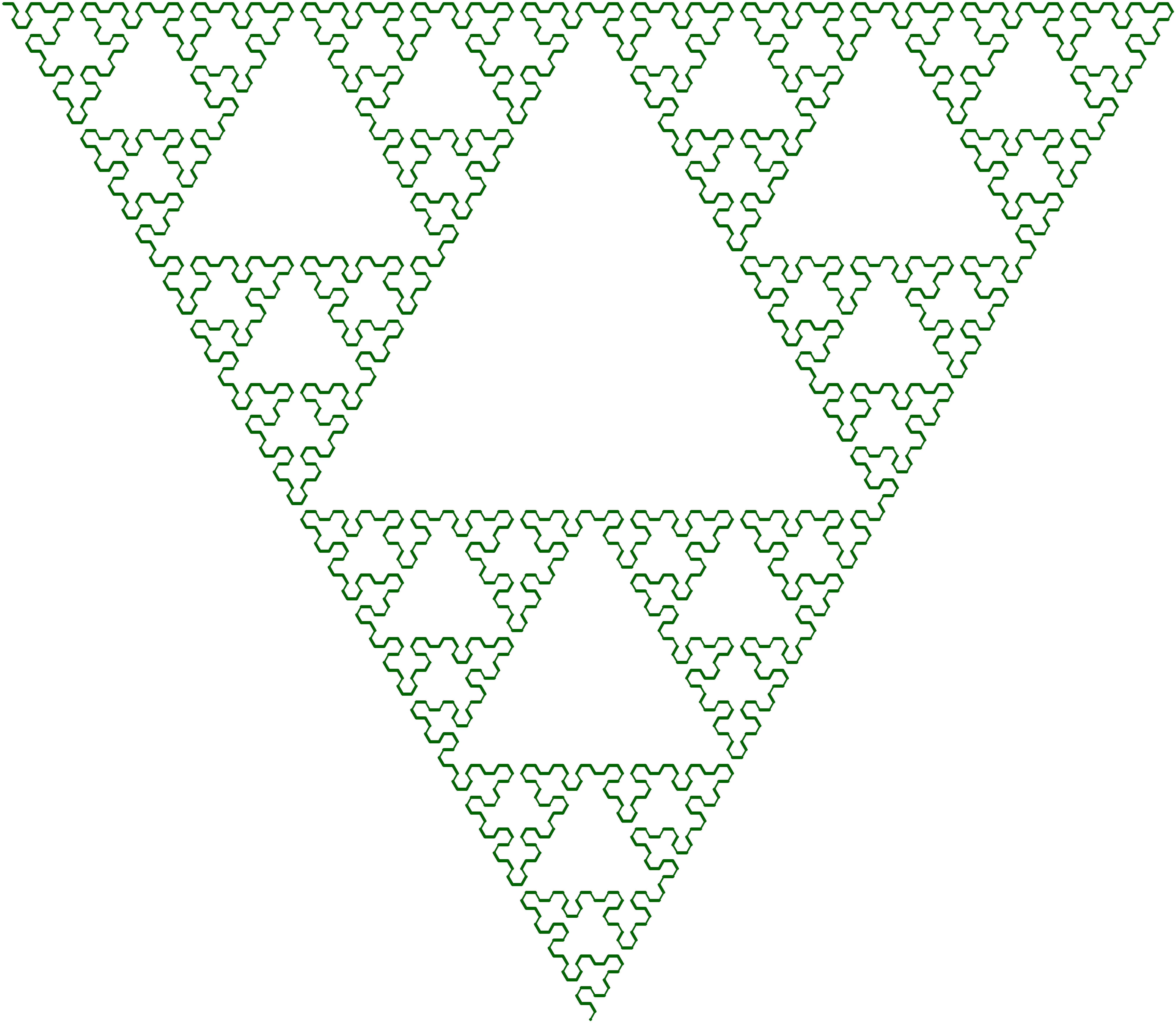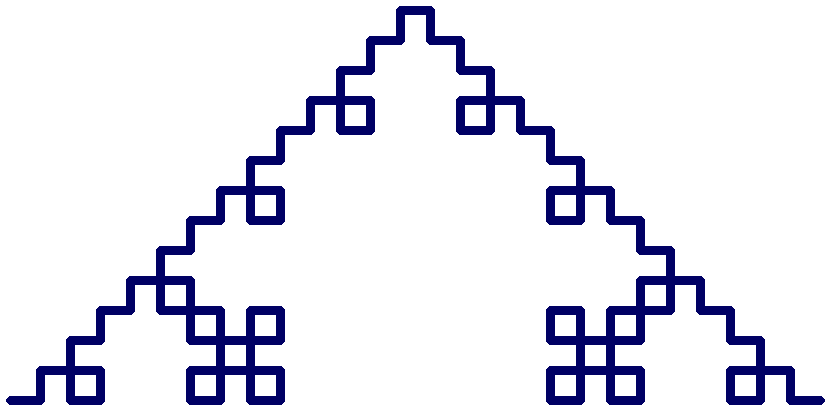## dcc-lsystem

An implementation of a Lindenmayer system together with some rendering tools

### 11 unstable releases

 0.6.3 May 17, 2021 Feb 10, 2020 Nov 2, 2019

#323 in Data structures

MIT/Apache and maybe AGPL-3.0-or-later

67KB
1K SLoC

# dcc-lsystem

A crate for working with Lindenmayer systems.

## Background

An L-System consists of an alphabet of symbols that can be used to make strings, a collection of production rules that expand each symbol into a larger string of symbols, an initial axiom string from which to begin construction, and a mechanism for transforming the generated strings into geometric structures.

## Algae example

Lindenmayer's original L-System for modelling the growth of Algae had variables `A` and `B`, axiom `A`, and production rules `A -> AB`, `B -> A`. Iterating this system produces the following output:

1. `A`
2. `AB`
3. `ABA`
4. `ABAAB`

## Basic usage

Put the following in your `Cargo.toml`:

``````dcc-lsystem = "0.6"
``````

### `LSystemBuilder`

An L-system is represented by an instance of `LSystem`. To create a barebones `LSystem`, the `LSystemBuilder` struct is useful. The following example shows an implementation of Lindenmayer's Algae system.

``````use dcc_lsystem::LSystemBuilder;

let mut builder = LSystemBuilder::new();

// Set up the two tokens we use for our system.
let a = builder.token("A");
let b = builder.token("B");

// Set up our axiom (i.e. initial state)
builder.axiom(vec![a]);

// Set the transformation rules
builder.transformation_rule(a, vec![a,b]); // A -> AB
builder.transformation_rule(b, vec![a]);   // B -> A

// Build our LSystem, which should have initial state A
let mut system = builder.finish();
assert_eq!(system.render(), "A");

// system.step() applies our production rules a single time
system.step();
assert_eq!(system.render(), "AB");

system.step();
assert_eq!(system.render(), "ABA");

// system.step_by() applies our production rule a number of times
system.step_by(5);
assert_eq!(system.render(), "ABAABABAABAABABAABABAABAABABAABAAB");
``````

## Rendering L-systems

It is possible to render an L-system into an image or gif. Typically this is done using a turtle - each token in the L-system's state is associated with some movement or rotation (or perhaps something more complicated) of a turtle. The `TurtleLSystemBuilder` struct offers a convenient way of constructing such renderings.

### Images

The Koch curve can be generated using an L-system with 3 symbols: `F`, `+`, and `-`, where `F` corresponds to moving forwards, `+` denotes a left rotation by 90°, and `-` denotes a right rotation by 90°. The system has axiom `F` and transformation rule `F => F+F-F-F+F`. This is implemented in the following example.

``````use image::Rgb;

use dcc_lsystem::turtle::{TurtleLSystemBuilder, TurtleAction};
use dcc_lsystem::renderer::{ImageRendererOptions, Renderer};

let mut builder = TurtleLSystemBuilder::new();

builder
.token("F", TurtleAction::Forward(30)) // F => go forward 30 units
.token("+", TurtleAction::Rotate(90))  // + => rotate left 90°
.token("-", TurtleAction::Rotate(-90)) // - => rotate right 90°
.axiom("F")
.rule("F => F + F - F - F + F");

let (mut system, renderer) = builder.finish();
system.step_by(5); // Iterate our L-system 5 times

let options = ImageRendererOptionsBuilder::new()
.thickness(4.0)
.fill_color(Rgb([255u8, 255u8, 255u8]))
.line_color(Rgb([0u8, 0u8, 100u8]))
.build();

renderer
.render(&system, &options)
.save("koch_curve.png")
.expect("Failed to save koch_curve.png");
``````

The resulting image is shown in the Examples section below.

### GIFs

It is also possible to render a GIF using an L-system. The individual frames of the GIF correspond to partial renderings of the L-system's state.

``````use image::Rgb;

use dcc_lsystem::renderer::{Renderer, VideoRendererOptions};
use dcc_lsystem::turtle::{TurtleAction, TurtleLSystemBuilder};

fn main() {
let mut builder = TurtleLSystemBuilder::new();

builder
.token("F", TurtleAction::Forward(30))
.token("+", TurtleAction::Rotate(90))
.token("-", TurtleAction::Rotate(-90))
.axiom("F")
.rule("F => F + F - F - F + F");

let (mut system, renderer) = builder.finish();
system.step_by(5);

let options = VideoRendererOptionsBuilder::new()
.filename("koch_curve.gif")
.fps(20)
.skip_by(0)
.thickness(4.0)
.fill_color(Rgb([255u8, 255u8, 255u8]))
.line_color(Rgb([0u8, 0u8, 100u8]))
.progress_bar(true)
.build();

renderer
.render(&system, &options);
}
``````

### Turtle actions

Currently the following actions are available:

`TurtleAction` Description
`Nothing` The turtle does nothing.
`Rotate(i32)` Rotate the turtle through an angle.
`Forward(i32)` Move the turtle forwards.
`Push` Push the turtle's current heading and location onto the stack.
`Pop` Pop the turtle's heading and location off the stack.
`StochasticRotate(Box<dyn Distribution>)` Rotate the turtle through an angle specified by some probability distribution.
`StochasticForward(Box<dyn Distribution>)` Move the turtle forwards through a distance specified by some probability distribution.

The `Distribution` trait is given by:

``````pub trait Distribution: dyn_clone::DynClone {
fn sample(&self) -> i32;
}
``````

A possible implementation of a Uniform distribution (using the `rand` crate) is as follows:

``````use rand::Rng;

#[derive(Clone)]
pub struct Uniform {
lower: i32,
upper: i32,
}

impl Uniform {
pub fn new(lower: i32, upper: i32) -> Self {
Self { lower, upper }
}
}

impl Distribution for Uniform {
fn sample(&self) -> i32 {
rng.gen_range(self.lower..=self.upper)
}
}
``````

## Examples

Examples are located in `dcc-lsystem/examples`.#### Koch curve#### Dragon curve#### Fractal plant